# Selina Solutions Concise Maths Class 10 Chapter 22 Heights and Distances

Trigonometry has a wide range of real-time applications and one among them is finding the heights and distances of buildings and between objects. This chapter is also one of the important chapters for ICSE Class 10. Understanding the angles of elevation and depression are the key fundamentals of this chapter. Students who find it difficult to solve problems of this chapter can access the Selina Solutions for Class 10 Mathematics prepared by subject matter experts at BYJU’S. This resource is created with an aim to boost confidence among students to take up their final board exams. The Selina Solutions for Class 10 Mathematics Chapter 22 Heights and Distances PDF are available exercise wise in the links attached below.

## Selina Solutions Concise Maths Class 10 Chapter 22 Heights and Distances Download PDF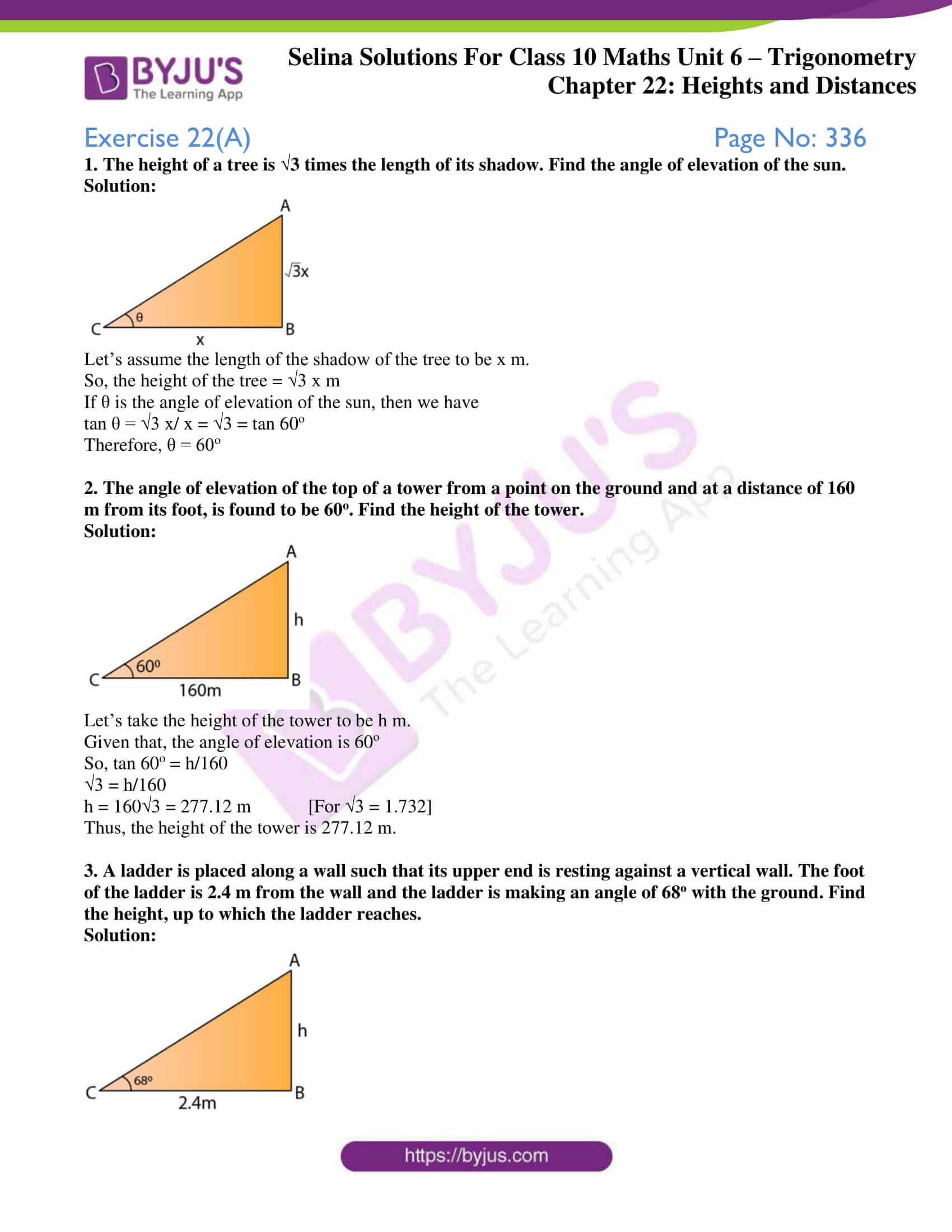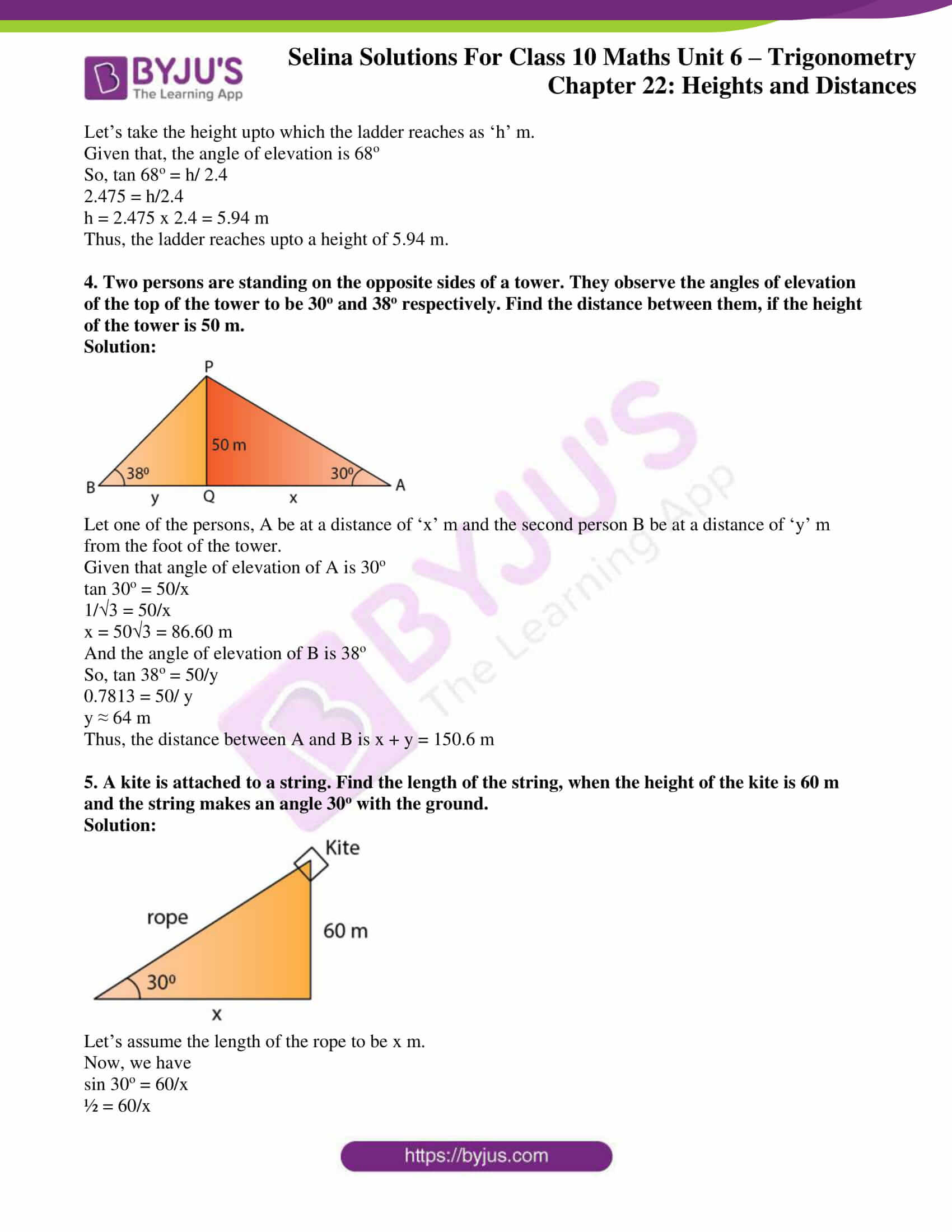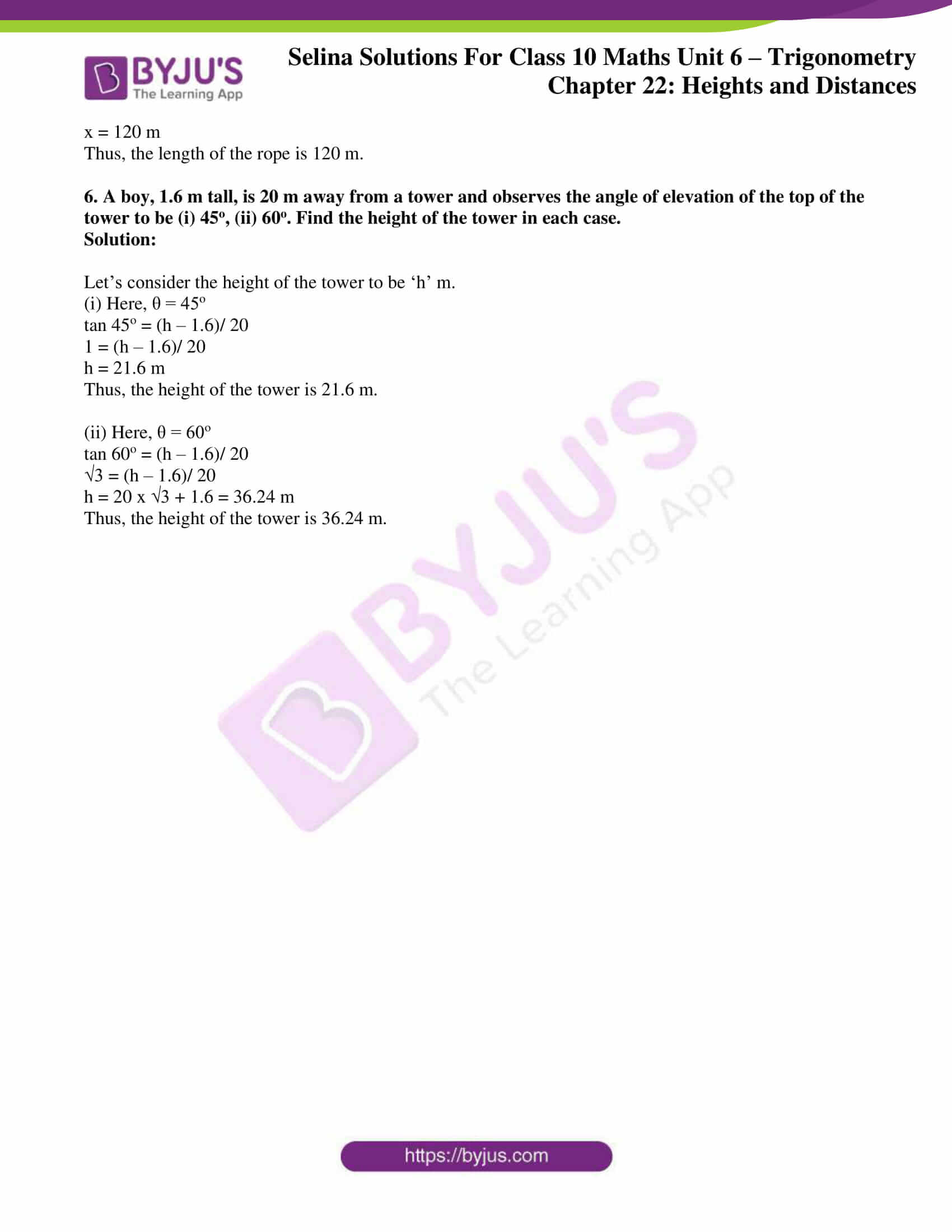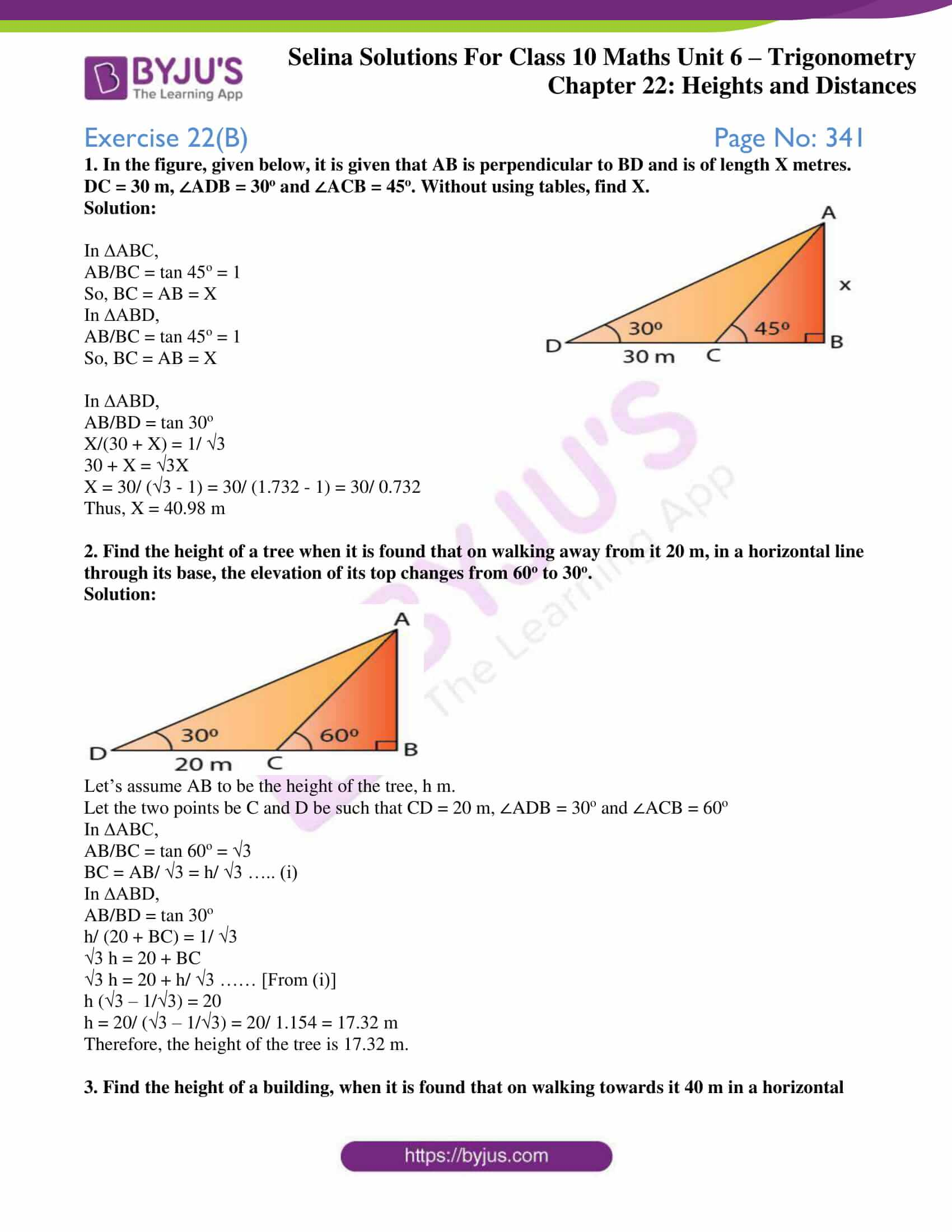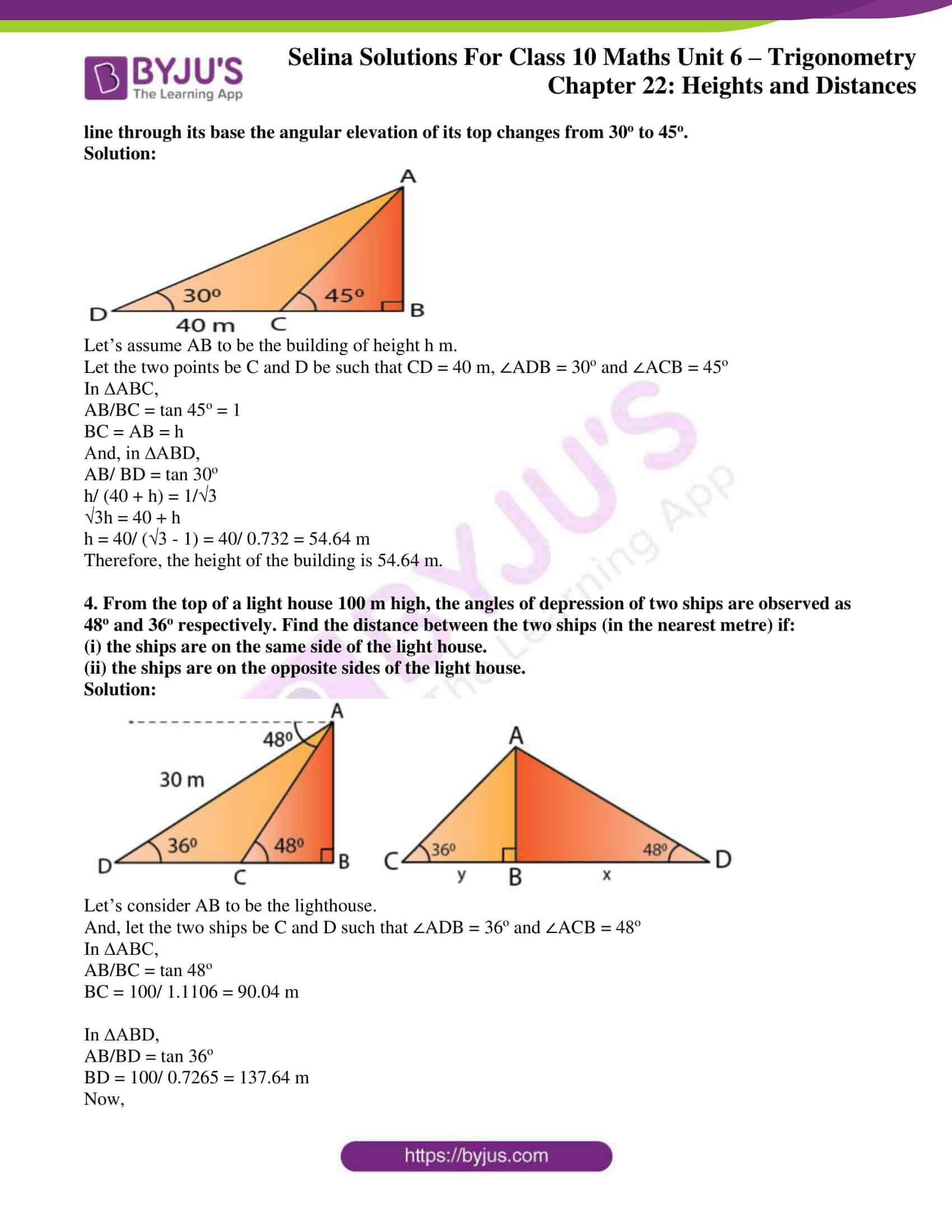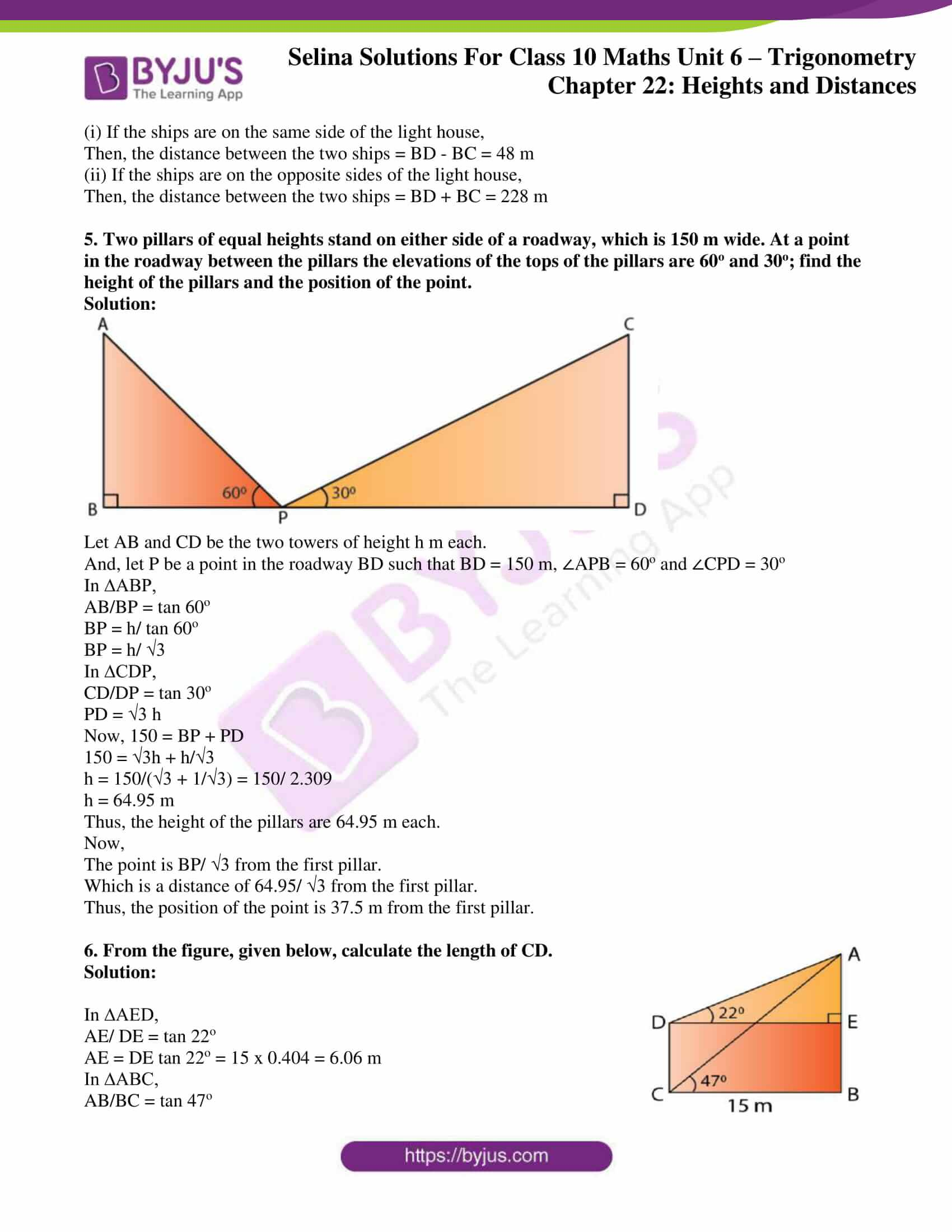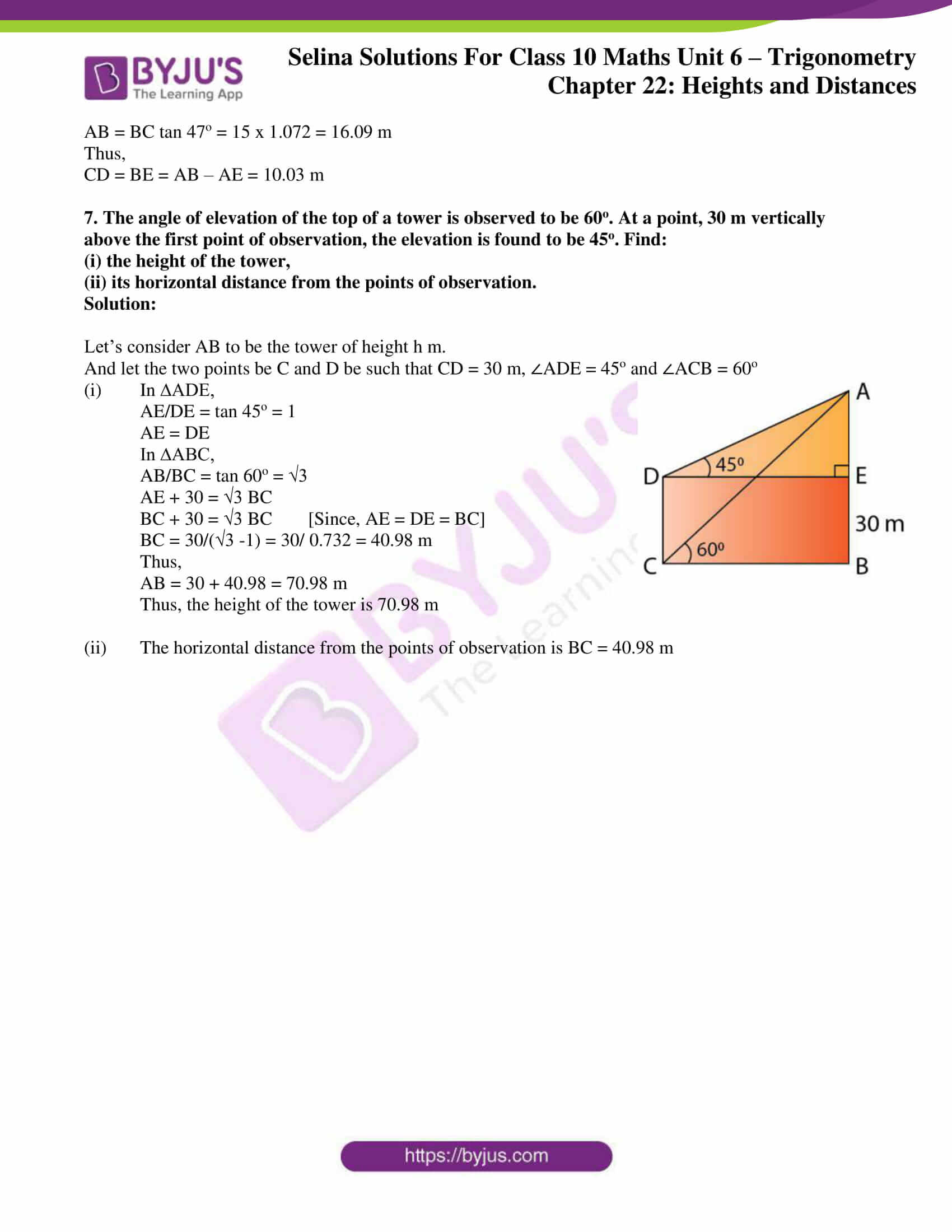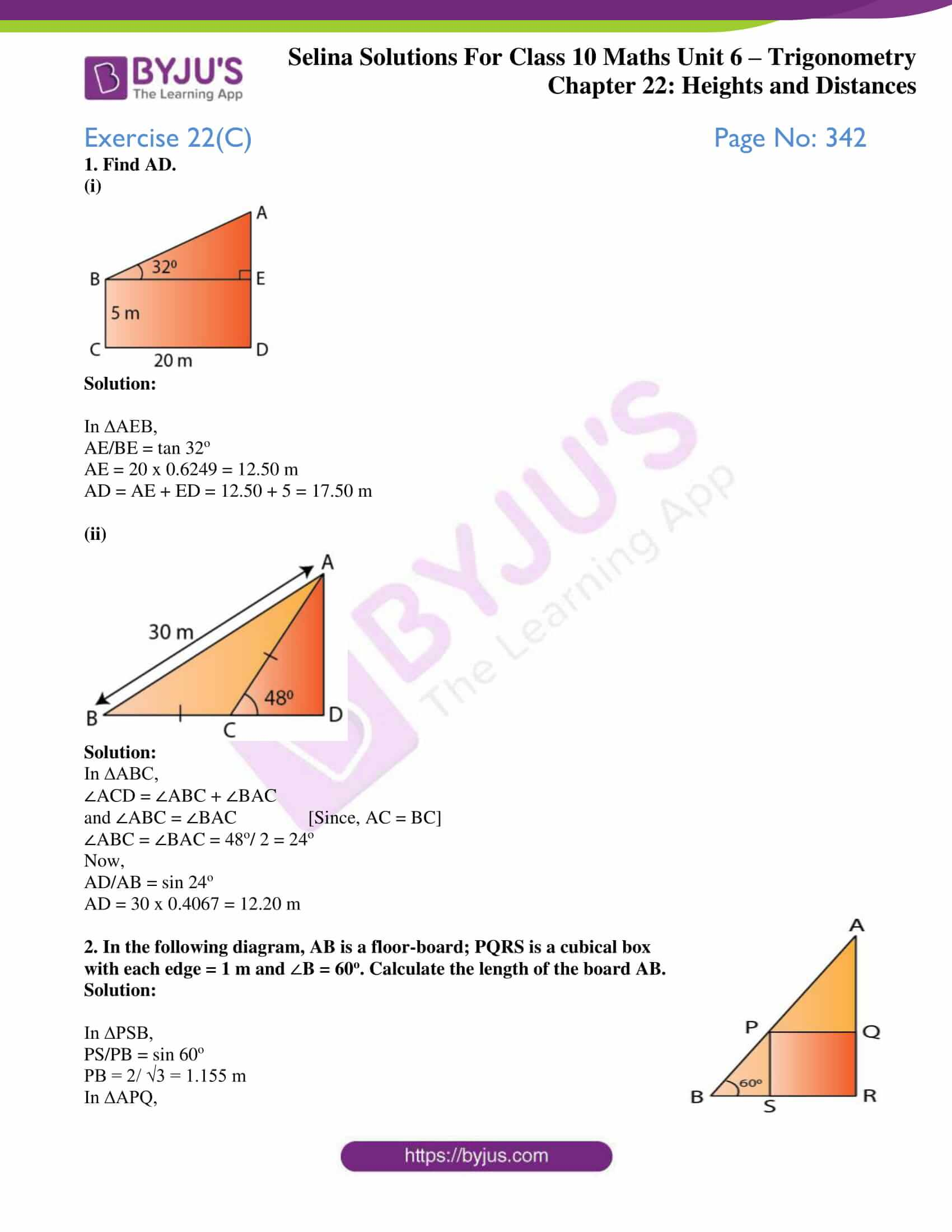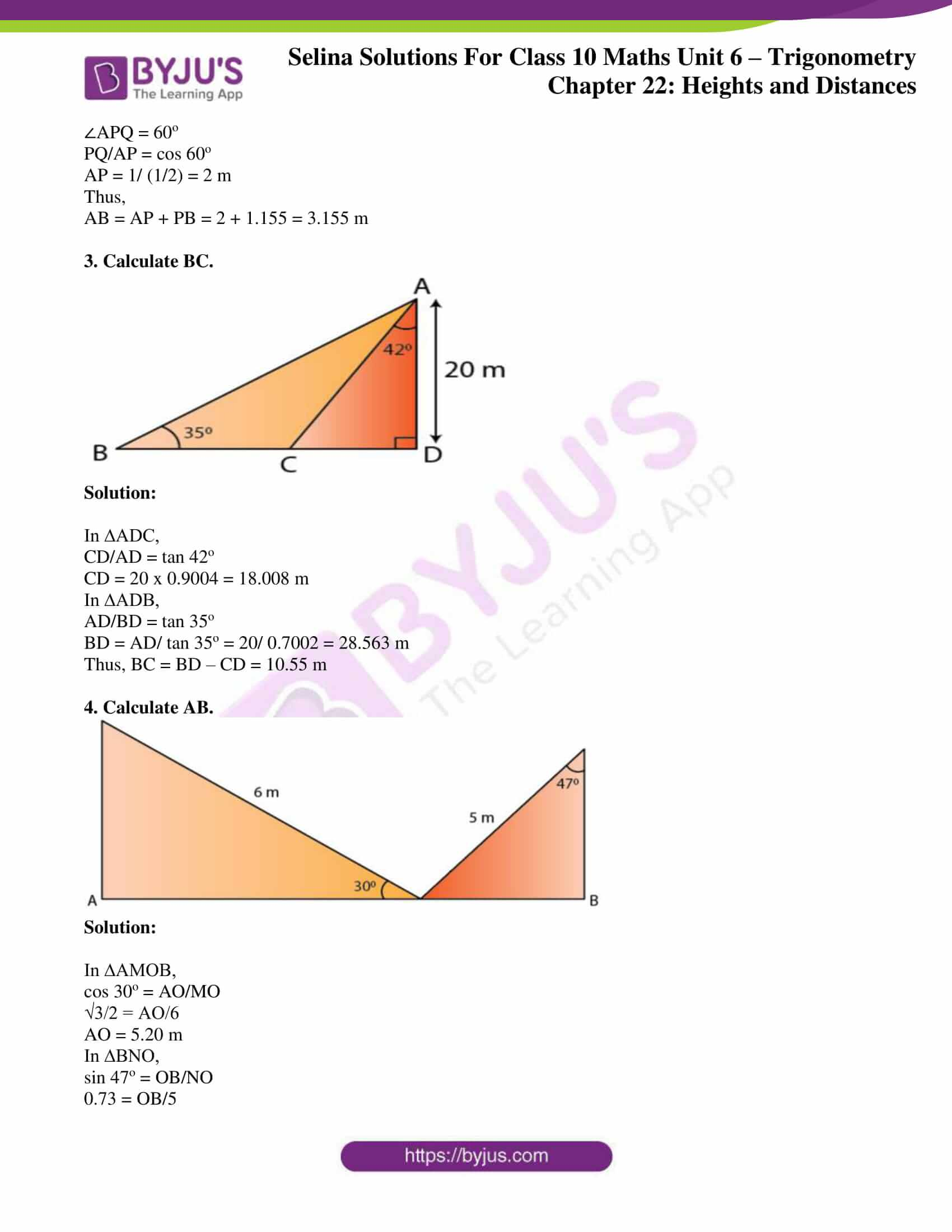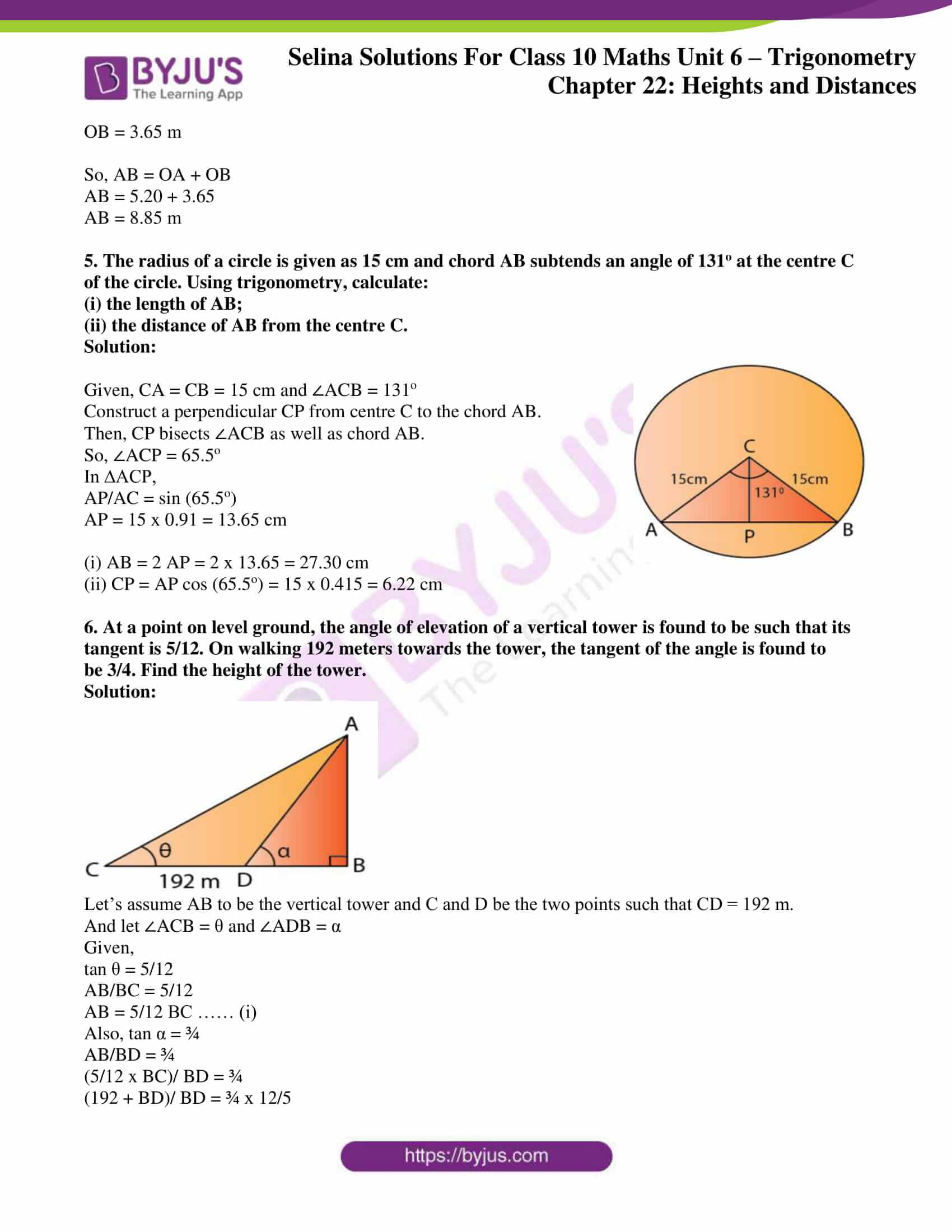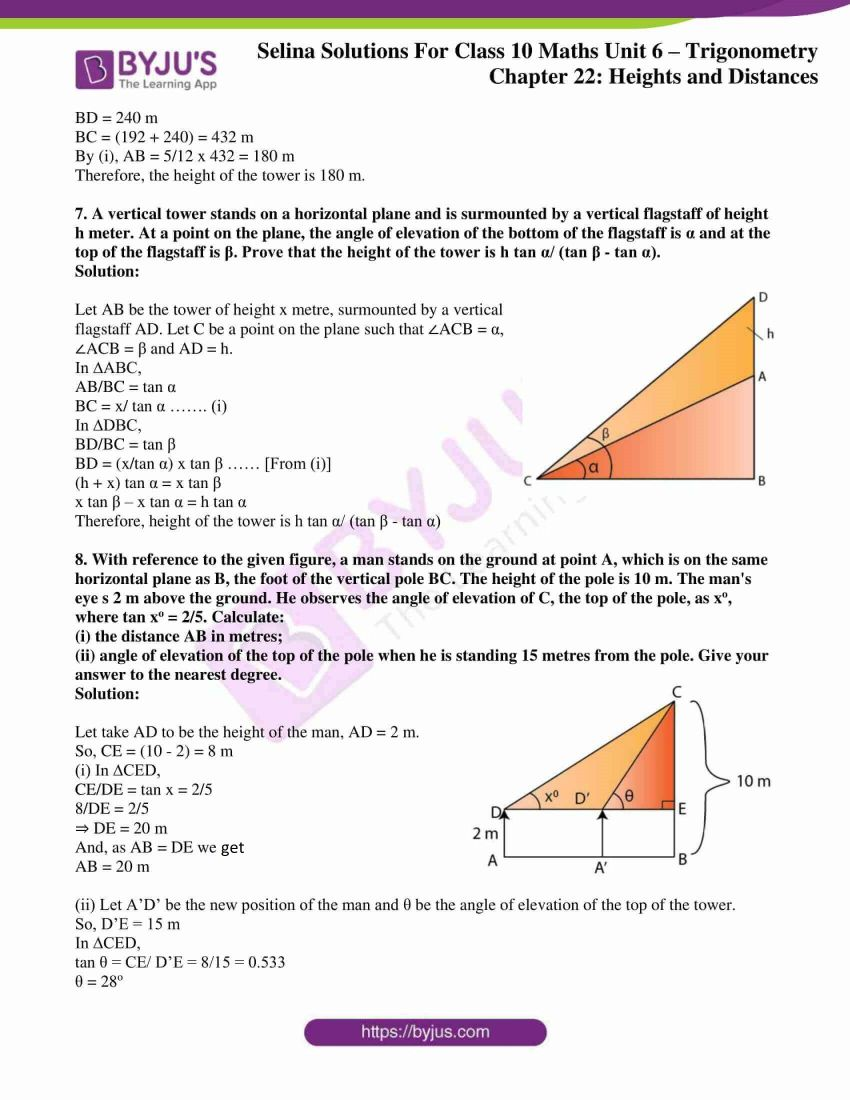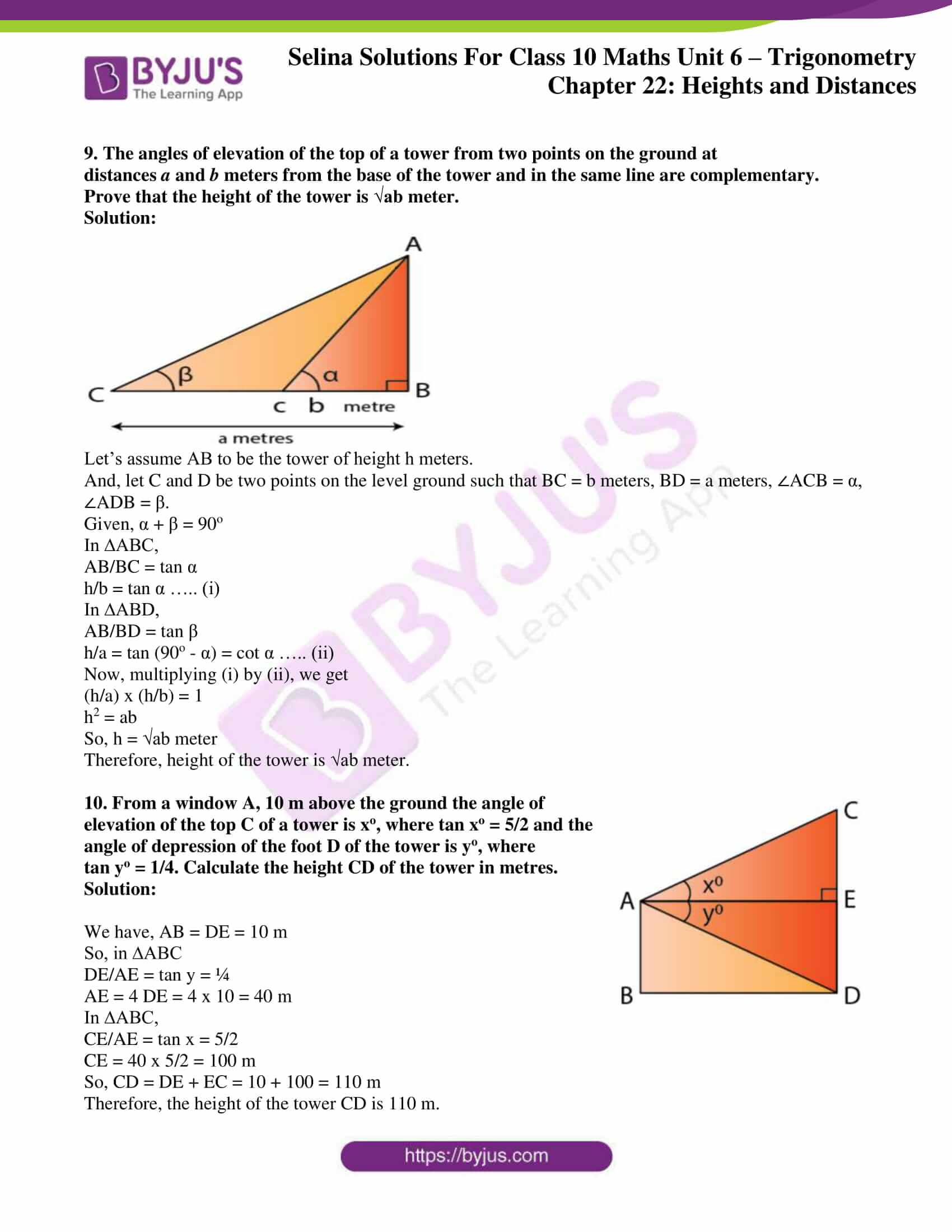### Exercises of Concise Selina Solutions Class 10 Maths Chapter 22 Heights and Distances

Exercise 22(A) Solutions

Exercise 22(B) Solutions

Exercise 22(C) Solutions

## Access Selina Solutions Concise Maths Class 10 Chapter 22 Heights and Distances

Exercise 22(A) Page No: 336

1. The height of a tree is √3 times the length of its shadow. Find the angle of elevation of the sun.

Solution: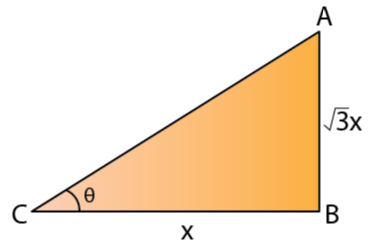Let’s assume the length of the shadow of the tree to be x m.

So, the height of the tree = √3 x m

If θ is the angle of elevation of the sun, then we have

tan θ = √3 x/ x = √3 = tan 60o

Therefore, θ = 60o

2. The angle of elevation of the top of a tower from a point on the ground and at a distance of 160 m from its foot, is found to be 60o. Find the height of the tower.

Solution: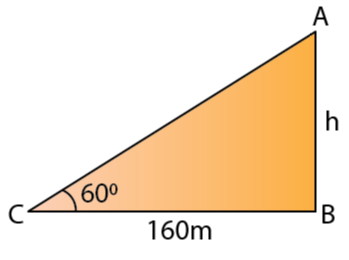Let’s take the height of the tower to be h m.

Given that, the angle of elevation is 60o

So, tan 60o = h/160

√3 = h/160

h = 160√3 = 277.12 m [For √3 = 1.732]

Thus, the height of the tower is 277.12 m.

3. A ladder is placed along a wall such that its upper end is resting against a vertical wall. The foot of the ladder is 2.4 m from the wall and the ladder is making an angle of 68o with the ground. Find the height, up to which the ladder reaches.

Solution: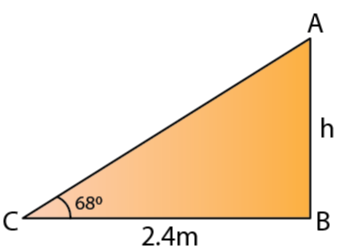Let’s take the height upto which the ladder reaches as ‘h’ m.

Given that, the angle of elevation is 68o

So, tan 68o = h/ 2.4

2.475 = h/2.4

h = 2.475 x 2.4 = 5.94 m

Thus, the ladder reaches upto a height of 5.94 m.

4. Two persons are standing on the opposite sides of a tower. They observe the angles of elevation of the top of the tower to be 30o and 38o respectively. Find the distance between them, if the height of the tower is 50 m.

Solution: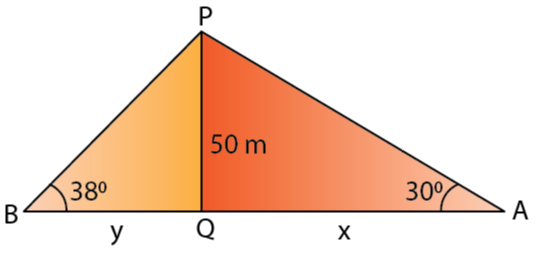Let one of the persons, A be at a distance of ‘x’ m and the second person B be at a distance of ‘y’ m from the foot of the tower.

Given that angle of elevation of A is 30o

tan 30o = 50/x

1/√3 = 50/x

x = 50√3 = 86.60 m

And the angle of elevation of B is 38o

So, tan 38o = 50/y

0.7813 = 50/ y

y ≈ 64 m

Thus, the distance between A and B is x + y = 150.6 m

5. A kite is attached to a string. Find the length of the string, when the height of the kite is 60 m and the string makes an angle 30o with the ground.

Solution: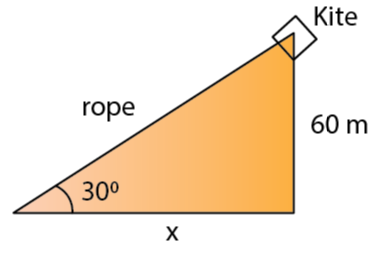Let’s assume the length of the rope to be x m.

Now, we have

sin 30o = 60/x

½ = 60/x

x = 120 m

Thus, the length of the rope is 120 m.

6. A boy, 1.6 m tall, is 20 m away from a tower and observes the angle of elevation of the top of the tower to be (i) 45o, (ii) 60o. Find the height of the tower in each case.

Solution:

Let’s consider the height of the tower to be ‘h’ m.

(i) Here, θ = 45o

tan 45o = (h – 1.6)/ 20

1 = (h – 1.6)/ 20

h = 21.6 m

Thus, the height of the tower is 21.6 m.

(ii) Here, θ = 60o

tan 60o = (h – 1.6)/ 20

√3 = (h – 1.6)/ 20

h = 20 x √3 + 1.6 = 36.24 m

Thus, the height of the tower is 36.24 m.

Exercise 22(B) Page No: 341

1. In the figure, given below, it is given that AB is perpendicular to BD and is of length X metres. DC = 30 m, ∠ADB = 30o and ∠ACB = 45o. Without using tables, find X.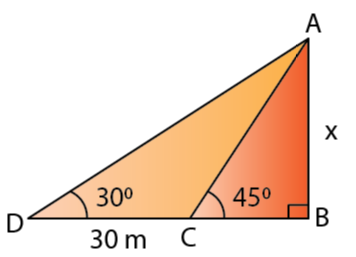Solution:

In ∆ABC,

AB/BC = tan 45o = 1

So, BC = AB = X

In ∆ABD,

AB/BC = tan 45o = 1

So, BC = AB = X

In ∆ABD,

AB/BD = tan 30o

X/(30 + X) = 1/ √3

30 + X = √3X

X = 30/ (√3 – 1) = 30/ (1.732 – 1) = 30/ 0.732

Thus, X = 40.98 m

2. Find the height of a tree when it is found that on walking away from it 20 m, in a horizontal line through its base, the elevation of its top changes from 60o to 30o.

Solution: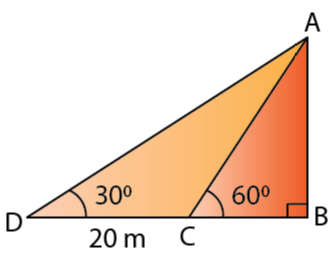Let’s assume AB to be the height of the tree, h m.

Let the two points be C and D be such that CD = 20 m, ∠ADB = 30o and ∠ACB = 60o

In ∆ABC,

AB/BC = tan 60o = √3

BC = AB/ √3 = h/ √3 ….. (i)

In ∆ABD,

AB/BD = tan 30o

h/ (20 + BC) = 1/ √3

√3 h = 20 + BC

√3 h = 20 + h/ √3 …… [From (i)]

h (√3 – 1/√3) = 20

h = 20/ (√3 – 1/√3) = 20/ 1.154 = 17.32 m

Therefore, the height of the tree is 17.32 m.

3. Find the height of a building, when it is found that on walking towards it 40 m in a horizontal line through its base the angular elevation of its top changes from 30o to 45o.

Solution: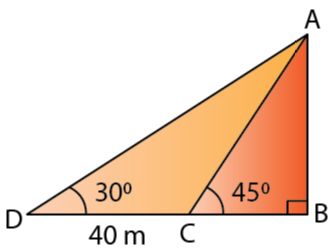Let’s assume AB to be the building of height h m.

Let the two points be C and D be such that CD = 40 m, ∠ADB = 30o and ∠ACB = 45o

In ∆ABC,

AB/BC = tan 45o = 1

BC = AB = h

And, in ∆ABD,

AB/ BD = tan 30o

h/ (40 + h) = 1/√3

√3h = 40 + h

h = 40/ (√3 – 1) = 40/ 0.732 = 54.64 m

Therefore, the height of the building is 54.64 m.

4. From the top of a light house 100 m high, the angles of depression of two ships are observed as 48o and 36o respectively. Find the distance between the two ships (in the nearest metre) if:

(i) the ships are on the same side of the light house.

(ii) the ships are on the opposite sides of the light house.

Solution: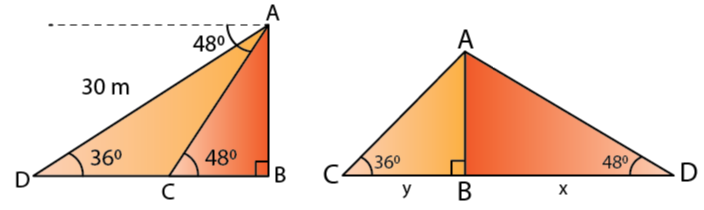Let’s consider AB to be the lighthouse.

And, let the two ships be C and D such that ∠ADB = 36o and ∠ACB = 48o

In ∆ABC,

AB/BC = tan 48o

BC = 100/ 1.1106 = 90.04 m

In ∆ABD,

AB/BD = tan 36o

BD = 100/ 0.7265 = 137.64 m

Now,

(i) If the ships are on the same side of the light house,

Then, the distance between the two ships = BD – BC = 48 m

(ii) If the ships are on the opposite sides of the light house,

Then, the distance between the two ships = BD + BC = 228 m

5. Two pillars of equal heights stand on either side of a roadway, which is 150 m wide. At a point in the roadway between the pillars the elevations of the tops of the pillars are 60o and 30o; find the height of the pillars and the position of the point.

Solution: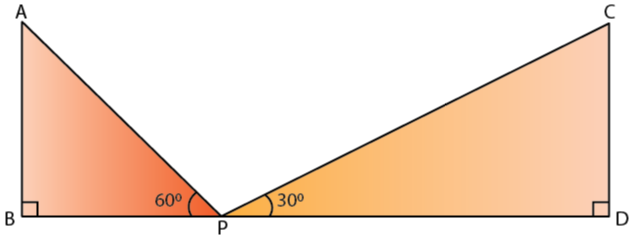Let AB and CD be the two towers of height h m each.

And, let P be a point in the roadway BD such that BD = 150 m, ∠APB = 60o and ∠CPD = 30o

In ∆ABP,

AB/BP = tan 60o

BP = h/ tan 60o

BP = h/ √3

In ∆CDP,

CD/DP = tan 30o

PD = √3 h

Now, 150 = BP + PD

150 = √3h + h/√3

h = 150/(√3 + 1/√3) = 150/ 2.309

h = 64.95 m

Thus, the height of the pillars are 64.95 m each.

Now,

The point is BP/ √3 from the first pillar.

Which is a distance of 64.95/ √3 from the first pillar.

Thus, the position of the point is 37.5 m from the first pillar.

6. From the figure, given below, calculate the length of CD.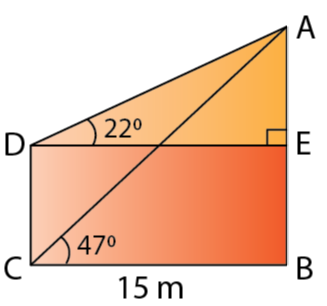Solution:

In ∆AED,

AE/ DE = tan 22o

AE = DE tan 22o = 15 x 0.404 = 6.06 m

In ∆ABC,

AB/BC = tan 47o

AB = BC tan 47o = 15 x 1.072 = 16.09 m

Thus,

CD = BE = AB – AE = 10.03 m

7. The angle of elevation of the top of a tower is observed to be 60o. At a point, 30 m vertically above the first point of observation, the elevation is found to be 45o. Find:

(i) the height of the tower,

(ii) its horizontal distance from the points of observation.

Solution:

Let’s consider AB to be the tower of height h m.

And let the two points be C and D be such that CD = 30 m, ∠ADE = 45o and ∠ACB = 60o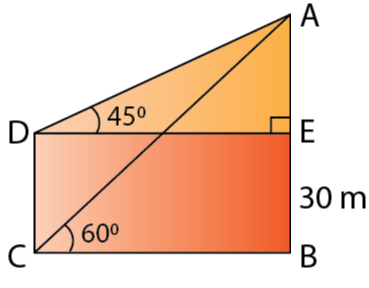(i) In ∆ADE,

AE/DE = tan 45o = 1

AE = DE

In ∆ABC,

AB/BC = tan 60o = √3

AE + 30 = √3 BC

BC + 30 = √3 BC [Since, AE = DE = BC]

BC = 30/(√3 -1) = 30/ 0.732 = 40.98 m

Thus,

AB = 30 + 40.98 = 70.98 m

Thus, the height of the tower is 70.98 m

(ii) The horizontal distance from the points of observation is BC = 40.98 m

Exercise 22(C) Page No: 342

(i)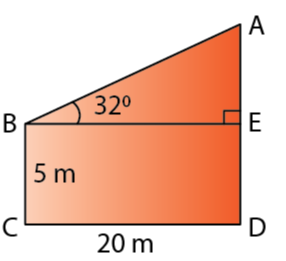Solution:

In ∆AEB,

AE/BE = tan 32o

AE = 20 x 0.6249 = 12.50 m

AD = AE + ED = 12.50 + 5 = 17.50 m

(ii)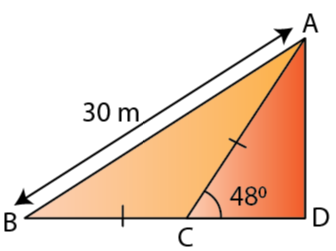Solution:

In ∆ABC,

∠ACD = ∠ABC + ∠BAC

and ∠ABC = ∠BAC [Since, AC = BC]

∠ABC = ∠BAC = 48o/ 2 = 24o

Now,

AD = 30 x 0.4067 = 12.20 m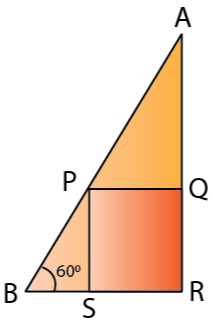2. In the following diagram, AB is a floor-board; PQRS is a cubical box with each edge = 1 m and B = 60o. Calculate the length of the board AB.

Solution:

In ∆PSB,

PS/PB = sin 60o

PB = 2/ √3 = 1.155 m

In ∆APQ,

∠APQ = 60o

PQ/AP = cos 60o

AP = 1/ (1/2) = 2 m

Thus,

AB = AP + PB = 2 + 1.155 = 3.155 m

3. Calculate BC.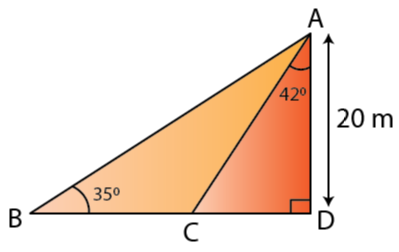Solution:

CD = 20 x 0.9004 = 18.008 m

BD = AD/ tan 35o = 20/ 0.7002 = 28.563 m

Thus, BC = BD – CD = 10.55 m

4. Calculate AB.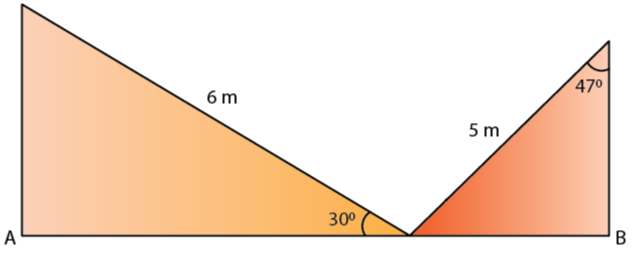Solution:

In ∆AMOB,

cos 30o = AO/MO

√3/2 = AO/6

AO = 5.20 m

In ∆BNO,

sin 47o = OB/NO

0.73 = OB/5

OB = 3.65 m

So, AB = OA + OB

AB = 5.20 + 3.65

AB = 8.85 m

5. The radius of a circle is given as 15 cm and chord AB subtends an angle of 131o at the centre C of the circle. Using trigonometry, calculate:

(i) the length of AB;

(ii) the distance of AB from the centre C.

Solution: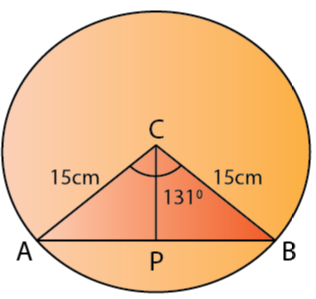Given, CA = CB = 15 cm and ∠ACB = 131o

Construct a perpendicular CP from centre C to the chord AB.

Then, CP bisects ∠ACB as well as chord AB.

So, ∠ACP = 65.5o

In ∆ACP,

AP/AC = sin (65.5o)

AP = 15 x 0.91 = 13.65 cm

(i) AB = 2 AP = 2 x 13.65 = 27.30 cm

(ii) CP = AP cos (65.5o) = 15 x 0.415 = 6.22 cm

6. At a point on level ground, the angle of elevation of a vertical tower is found to be such that its tangent is 5/12. On walking 192 meters towards the tower, the tangent of the angle is found to be 3/4. Find the height of the tower.

Solution: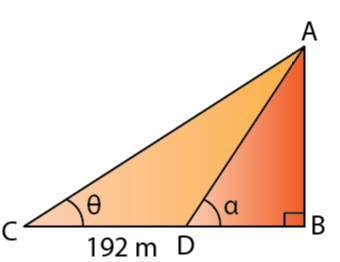Let’s assume AB to be the vertical tower and C and D be the two points such that CD = 192 m.

And let ∠ACB = θ and ∠ADB = α

Given,

tan θ = 5/12

AB/BC = 5/12

AB = 5/12 BC …… (i)

Also, tan α = ¾

AB/BD = ¾

(5/12 x BC)/ BD = ¾

(192 + BD)/ BD = ¾ x 12/5

BD = 240 m

BC = (192 + 240) = 432 m

By (i), AB = 5/12 x 432 = 180 m

Therefore, the height of the tower is 180 m.

7. A vertical tower stands on a horizontal plane and is surmounted by a vertical flagstaff of height h meter. At a point on the plane, the angle of elevation of the bottom of the flagstaff is α and at the top of the flagstaff is β. Prove that the height of the tower is h tan α/ (tan β – tan α).

Solution: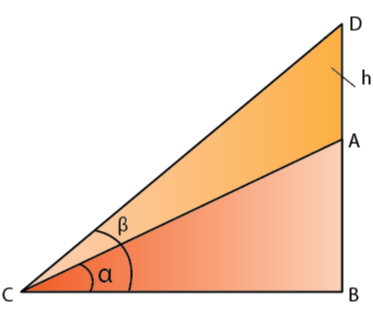Let AB be the tower of height x metre, surmounted by a vertical flagstaff AD. Let C be a point on the plane such that ∠ACB = α, ∠ACB = β and AD = h.

In ∆ABC,

AB/BC = tan α

BC = x/ tan α ……. (i)

In ∆DBC,

BD/BC = tan β

BD = (x/tan α) x tan β …… [From (i)]

(h + x) tan α = x tan β

x tan β – x tan α = h tan α

Therefore, height of the tower is h tan α/ (tan β – tan α)

8. With reference to the given figure, a man stands on the ground at point A, which is on the same horizontal plane as B, the foot of the vertical pole BC. The height of the pole is 10 m. The man’s eye s 2 m above the ground. He observes the angle of elevation of C, the top of the pole, as xo, where tan xo = 2/5. Calculate:

(i) the distance AB in metres;

(ii) angle of elevation of the top of the pole when he is standing 15 metres from the pole. Give your answer to the nearest degree.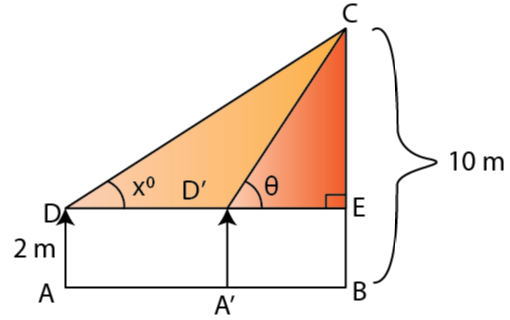Solution:

Let take AD to be the height of the man, AD = 2 m.

So, CE = (10 – 2) = 8 m

(i) In ∆CED,

CE/DE = tan x = 2/5

8/DE = 2/5

⇒ DE = 20 m

And, as AB = DE we get,

AB = 20 m

(ii) Let A’D’ be the new position of the man and θ be the angle of elevation of the top of the tower.

So, D’E = 15 m

In ∆CED,

tan θ = CE/ D’E = 8/15 = 0.533

θ = 28o

9. The angles of elevation of the top of a tower from two points on the ground at distances a and b meters from the base of the tower and in the same line are complementary. Prove that the height of the tower is √ab meter.

Solution: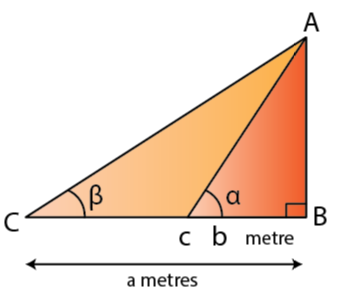Let’s assume AB to be the tower of height h meters.

And, let C and D be two points on the level ground such that BC = b meters, BD = a meters, ∠ACB = α, ∠ADB = β.

Given, α + β = 90o

In ∆ABC,

AB/BC = tan α

h/b = tan α ….. (i)

In ∆ABD,

AB/BD = tan β

h/a = tan (90o – α) = cot α ….. (ii)

Now, multiplying (i) by (ii), we get

(h/a) x (h/b) = 1

h2 = ab

So, h = √ab meter

Therefore, height of the tower is √ab meter.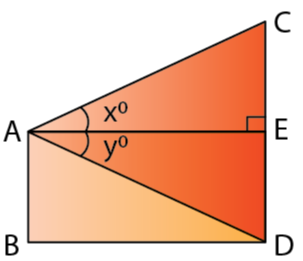10. From a window A, 10 m above the ground the angle of elevation of the top C of a tower is xo, where tan xo = 5/2 and the angle of depression of the foot D of the tower is yo, where tan yo = 1/4. Calculate the height CD of the tower in metres.

Solution:

We have, AB = DE = 10 m

So, in ∆ABC

DE/AE = tan y = ¼

AE = 4 DE = 4 x 10 = 40 m

In ∆ABC,

CE/AE = tan x = 5/2

CE = 40 x 5/2 = 100 m

So, CD = DE + EC = 10 + 100 = 110 m

Therefore, the height of the tower CD is 110 m.

The given solutions are as per the 2019-20 Concise Selina textbook. The Selina Solutions for the academic year 2020-21 will be updated soon.